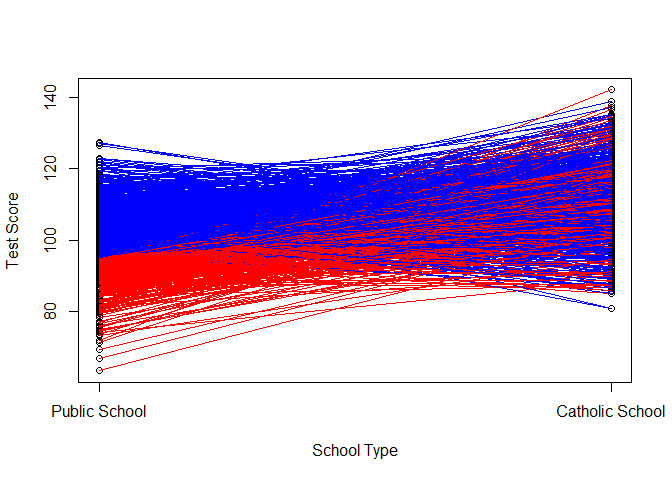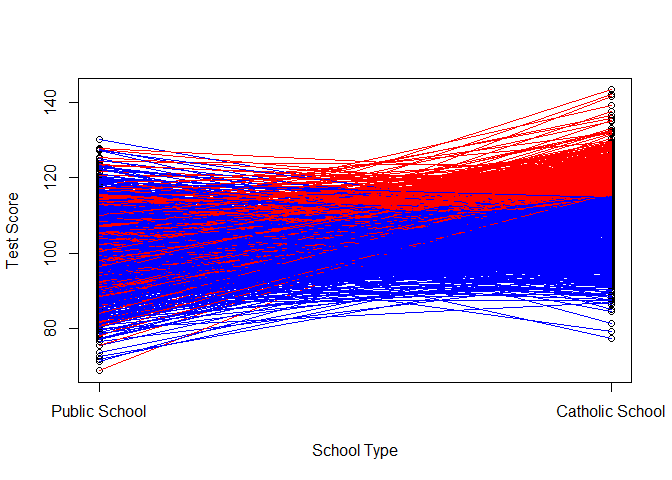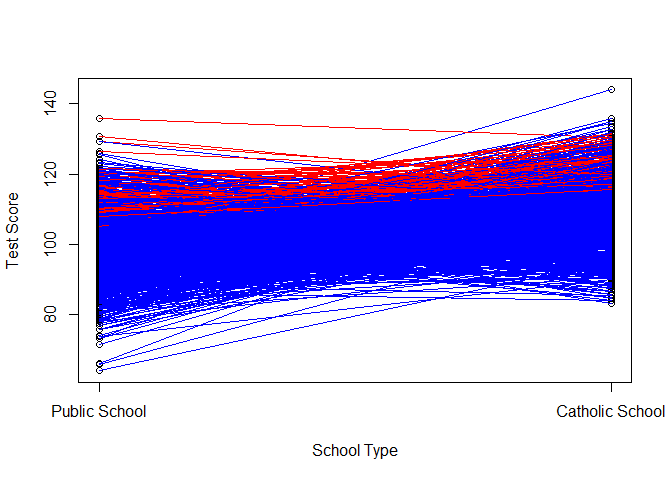+++ date = 2018-02-19 # lastmod = 2018-02-19 draft = false tags = [“potential outcomes”, “causal”, “regression”, “endogenous selection bias”] title = “Regression and Potential Outcomes” summary = “What follows is an example I worked up when trying to figure out how to communicate potential outcomes in a regression framework to students graphically. This discussion is derived from Morgan and Winship’s 2015 book, especially pages 122-123. The goal is to represent the potential outcomes framework using standard regression notation, and then to discuss endogenous selection bias using this framework.” +++

## Introduction

What follows is an example I worked up when trying to figure out how to communicate potential outcomes in a regression framework to students graphically. This discussion is derived from Morgan and Winship’s 2015 book1, especially pages 122-123. The goal is to represent the potential outcomes framework using standard regression notation, and then to discuss endogenous selection bias using this framework.

## Math

Define Yiâ=âÎ¼0â+â(Î¼1âââÎ¼0)Diâ+â{vi0â+âDi(vi1âââvi0)} where Di is binary assignment to treatment for individual i, Î¼0 is the expected outcome for control, Î¼1 is the expected outcome for treated, Î¼1âââÎ¼0 is the Average Treatment Effect or Î´. The v terms are subset by i denoting that they are individual heterogeneity. vi0 is an individual’s deviation from Î¼0 under control, or Yi0â=âÎ¼0â+âvi0. The same can be said for vi1, it is an individuals deviation from Î¼1 under treatment or Yi1â=âÎ¼1â+âvi1. Treatment minus control is equal to (Yi1)â(Yi0)=(Î¼1â+âvi1)â(Î¼0â+âvi0) which is equal to the average treatment effect Î¼1âââÎ¼0 and the individual heterogeneity in the treatment effect vi1âââvi0.

For Î¼0 to properly represent the mean of Yi under control, the E$*v*<sub>*i*</sub><sup>0</sup>$ must be equal to zero, or $$\\dfrac{\\Sigma\_i^N v^0\_i}{N} = \\Sigma\_i^n v^0\_i= 0$$. You can get here from the linearity of expectation, such that if Î¼0â=âE$*Y*<sub>*i*</sub>$ for control, we can substitute such that Î¼0â=âE$*Î¼*<sub>0</sub>â +â *v*<sub>*i*</sub><sup>0</sup>$=E$*Î¼*<sub>0</sub>$+E$*v*<sub>*i*</sub><sup>0</sup>$=Î¼0â+âE$*v*<sub>*i*</sub><sup>0</sup>$, therefore E$*v*<sub>*i*</sub><sup>0</sup>$=0. The same is true for E$*v*<sub>*i*</sub><sup>1</sup>$, Î¼1â=âE$*Î¼*<sub>1</sub>â +â *v*<sub>*i*</sub><sup>1</sup>$=E$*Î¼*<sub>1</sub>$+E$*v*<sub>*i*</sub><sup>1</sup>$=Î¼1â+âE$*v*<sub>*i*</sub><sup>1</sup>$. By the same token, then E$*v*<sub>*i*</sub><sup>1</sup>â ââ *v*<sub>*i*</sub><sup>0</sup>$=E$*v*<sub>*i*</sub><sup>1</sup>$âE$*v*<sub>*i*</sub><sup>0</sup>$=0.

Morgan and Winship argue that issues arise when “Di is correlated with the population-level variant of the error term, {vi0â+âDi(vi1âââvi0)}, as would be the case when the size of the individual-level treatment effect, in this case (Î¼1âââÎ¼0)+{vi0â+âDi(vi1âââvi0)}, differs among those who select the treatment and those who do not.” For E$(*Î¼*<sub>1</sub>â ââ *Î¼*<sub>0</sub>)+{*v*<sub>*i*</sub><sup>0</sup>â +â *D*<sub>*i*</sub>(*v*<sub>*i*</sub><sup>1</sup>â ââ *v*<sub>*i*</sub><sup>0</sup>)}|*D*<sub>*i*</sub>â=â1$ to be greater than E$(*Î¼*<sub>1</sub>â ââ *Î¼*<sub>0</sub>)+{*v*<sub>*i*</sub><sup>0</sup>â +â *D*<sub>*i*</sub>(*v*<sub>*i*</sub><sup>1</sup>â ââ *v*<sub>*i*</sub><sup>0</sup>)}|*D*<sub>*i*</sub>â=â0$, we need to have E$*v*<sub>1</sub><sup>1</sup>|*D*<sub>*i*</sub>â=â1$>E$*v*<sub>1</sub><sup>0</sup>|*D*<sub>*i*</sub>â=â0$ which does not imply E$*v*<sub>*i*</sub><sup>1</sup>$>E$*v*<sub>*i*</sub><sup>0</sup>$ which would have violated our previous statements. If E$*v*<sub>*i*</sub><sup>1</sup>|*D*<sub>*i*</sub>â=â1$=E$*v*<sub>*i*</sub><sup>1</sup>$ and E$*v*<sub>*i*</sub><sup>0</sup>|*D*<sub>*i*</sub>â=â0$=E$*v*<sub>*i*</sub><sup>0</sup>$ as with random assignment, we can get an unbiased estimate of Î´ with a simple regression of Yi on Di. When E$*v*<sub>*i*</sub><sup>1</sup>|*D*<sub>*i*</sub>â=â1$â E$*v*<sub>*i*</sub><sup>1</sup>$ and/or E$*v*<sub>*i*</sub><sup>0</sup>|*D*<sub>*i*</sub>â=â0$â E$*v*<sub>*i*</sub><sup>0</sup>$, as when Di is assigned to those with high individual treatment effects, we cannot get an unbiased estimate of Î´ with a simple regression.

## Worked Examples (Code in R)

Take, for example, the case of catholic school (following Morgan and Winships examples). Assume for the moment that the average test score of a public school student is equal to 100, such that Î¼0â=â100, and the average test score of a catholic school student is equal to 110, such that Î¼1â=â110. The Average Treatment Effect, Î´, equals Î¼1âââÎ¼0â=â10. Next, assume random variability in test performance such that v1 and v0 follow a normal distribution with mean zero and variance 100. Let this represent individual level variability in test taking. A graph of potential outcomes for students under different school types is shown below. The outcomes for a single individual, i, are connected with a line.If we first assume selection into catholic school or public school (i.e. Di is randomly assigned), we would no correlation between treatment and errors, and our simple regression correctly estimates the Average Treatment Effect. See below.

mu0 <- 100
mu1 <- 110
v0 <- rnorm(1000, mean=0, sd=10)
v1 <- rnorm(1000, mean=0, sd=10)
d <- rbinom(1000,1,.5)
y <- mu0 + (mu1 - mu0)*d + v0+d*(v1-v0)
mean(y[d==1])

##  110.0734

mean(y[d==0])

##  99.81277

summary(lm(y~d))

##
## Call:
## lm(formula = y ~ d)
##
## Residuals:
##     Min      1Q  Median      3Q     Max
## -30.639  -7.357  -0.213   7.181  39.384
##
## Coefficients:
##             Estimate Std. Error t value Pr(>|t|)
## (Intercept)  99.8128     0.4478  222.90   <2e-16 ***
## d            10.2606     0.6581   15.59   <2e-16 ***
## ---
## Signif. codes:  0 '***' 0.001 '**' 0.01 '*' 0.05 '.' 0.1 ' ' 1
##
## Residual standard error: 10.38 on 998 degrees of freedom
## Multiple R-squared:  0.1959, Adjusted R-squared:  0.1951
## F-statistic: 243.1 on 1 and 998 DF,  p-value: < 2.2e-16

cor(d, v1)

##  0.01767286

cor(d, v0)

##  0.008900606

cor(d, (v1-v0))

##  0.006432687

A graphical representation is below. Red lines represent kids in catholic school, blue lines represent kids in public school. Note: our observational data would only include those scores for red dots in catholic school and blue dots in public school.Say, instead, that public school kids with a history of bad test scores are selected to attend catholic school. Their expected v0 is less than negative 5. Further assume that these kids only under perform in public school such that we would not expect their average deviations from E$*v*<sub>*i*</sub><sup>1</sup>$ to be biased, or E$*v*<sub>*i*</sub><sup>1</sup>|*D*â=â1$=0. We’ve now selected out the low performers from the public school population, increasing the baseline public school test scores, and left the catholic school test scores unchanged. Further, we’ve induced a correlation between Di and vi0 and between Di and vi1âââvi0 but not between Di and vi1. The estimated causal effect would be lower than the average treatment effect for this scenario. See below for the worked example.

mu0 <- 100
mu1 <- 110
v0 <- rnorm(1000, mean=0, sd=10)
v1 <- rnorm(1000, mean=0, sd=10)
d <- v0< -5
y <- mu0 + (mu1 - mu0)*d + v0+d*(v1-v0)
mean(y[d==1])

##  110.5723

mean(y[d==0])

##  104.9255

summary(lm(y~d))

##
## Call:
## lm(formula = y ~ d)
##
## Residuals:
##      Min       1Q   Median       3Q      Max
## -25.0500  -6.0554  -0.8261   4.8245  26.4238
##
## Coefficients:
##             Estimate Std. Error t value Pr(>|t|)
## (Intercept) 104.9255     0.3108  337.63   <2e-16 ***
## dTRUE         5.6467     0.5394   10.47   <2e-16 ***
## ---
## Signif. codes:  0 '***' 0.001 '**' 0.01 '*' 0.05 '.' 0.1 ' ' 1
##
## Residual standard error: 8.032 on 998 degrees of freedom
## Multiple R-squared:  0.09896,    Adjusted R-squared:  0.09806
## F-statistic: 109.6 on 1 and 998 DF,  p-value: < 2.2e-16

cor(d, v1)

##  0.02248338

cor(d, v0)

##  -0.7704901

cor(d, (v1-v0))

##  0.5620342

If we were to graph the potential outcomes, we would see the following:We can do the same for an example where we only select kids who we think will prosper in catholic school for treatment. We only choose those who have a vi1â>â5, but we ignore vi0 in our treatment assignment. We have left the baseline performance unchanged (we’ve randomly selected on vi0 after all), but we’ve increased the performance of catholic school children on average. We’ve thus induced a correlation between Di and vi1 and between Di and vi1âââvi0 but not between Di and vi0. See below for the worked example.

mu0 <- 100
mu1 <- 110
v0 <- rnorm(1000, mean=0, sd=10)
v1 <- rnorm(1000, mean=0, sd=10)
d <- v1 > 5
y <- mu0 + (mu1 - mu0)*d + v0+d*(v1-v0)
mean(y[d==1])

##  121.4591

mean(y[d==0])

##  99.93334

summary(lm(y~d))

##
## Call:
## lm(formula = y ~ d)
##
## Residuals:
##      Min       1Q   Median       3Q      Max
## -27.9563  -5.4148  -0.4595   5.8804  29.9768
##
## Coefficients:
##             Estimate Std. Error t value Pr(>|t|)
## (Intercept)  99.9333     0.3439  290.62   <2e-16 ***
## dTRUE        21.5258     0.6107   35.24   <2e-16 ***
## ---
## Signif. codes:  0 '***' 0.001 '**' 0.01 '*' 0.05 '.' 0.1 ' ' 1
##
## Residual standard error: 8.987 on 998 degrees of freedom
## Multiple R-squared:  0.5545, Adjusted R-squared:  0.5541
## F-statistic:  1242 on 1 and 998 DF,  p-value: < 2.2e-16

cor(d, v1)

##  0.7707782

cor(d, v0)

##  0.02700884

cor(d, (v1-v0))

##  0.509484

The plot of this example would look like this:We can also select those kids who would do well in either catholic school or in public school. Say we assign treatment to those with vi0 and vi1 greater than 5. We’ve thus pushed down the public school scores while increasing the catholic school scores. We’ve induced a correlation between Di and both vi0 and vi1 but not between Di and vi1âââvi0. See below for the worked example.

mu0 <- 100
mu1 <- 110
v0 <- rnorm(1000, mean=0, sd=10)
v1 <- rnorm(1000, mean=0, sd=10)
d <- v0 > 10 & v1 > 5
y <- mu0 + (mu1 - mu0)*d + v0+d*(v1-v0)
mean(y[d==1])

##  121.842

mean(y[d==0])

##  98.6236

summary(lm(y~d))

##
## Call:
## lm(formula = y ~ d)
##
## Residuals:
##     Min      1Q  Median      3Q     Max
## -26.732  -6.639  -0.066   6.724  32.485
##
## Coefficients:
##             Estimate Std. Error t value Pr(>|t|)
## (Intercept)  98.6236     0.3127  315.40   <2e-16 ***
## dTRUE        23.2184     1.3713   16.93   <2e-16 ***
## ---
## Signif. codes:  0 '***' 0.001 '**' 0.01 '*' 0.05 '.' 0.1 ' ' 1
##
## Residual standard error: 9.628 on 998 degrees of freedom
## Multiple R-squared:  0.2232, Adjusted R-squared:  0.2224
## F-statistic: 286.7 on 1 and 998 DF,  p-value: < 2.2e-16

cor(d, v1)

##  0.2733187

cor(d, v0)

##  0.3571695

cor(d, (v1-v0))

##  -0.06421799

And the plot:## End Notes

Thanks to Monica Alexander who looked over an early draft.

Morgan, Stephen L. and Christopher Winship. 2015. Counterfactuals and Causal Inference. Methods and Principles for Social Research. Second Edition. Cambridge University Press.